# Ring Modulator for The Double Sideband Suppressed Carrier Generation

## Diode Ring Modulator

Fig.1 shows the circuit diagram of a diode ring modulator .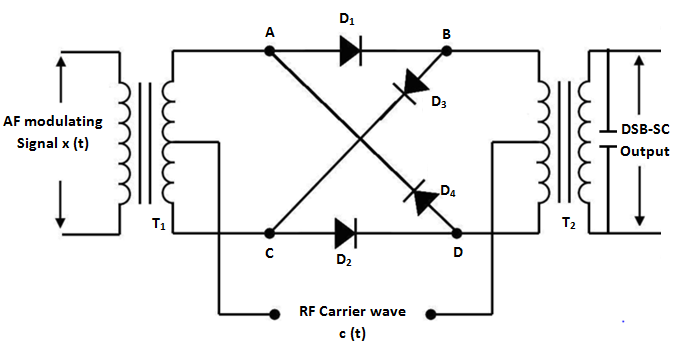Fig. 1 : Ring Modulator

It consists of four diodes, an audio frequency transformer Tand an RF transformer T.

The carrier signal is assumed to be a square wave with frequency fc  and it is connected between the centre taps of the two transformers .

The DSB-SC output is obtained at the secondary of the RF transformer T.

## Working Operation

The operation of the ring modulator is explained with the assumptions that the diodes act as perfect switches and that they are switched ON  and OFF by the RF carrier signal . This is because the amplitude and frequency of the carrier is higher than that of the modulating signal .

The operation can be divided into different modes without the modulating signal and with the modulating signal as follows :

### Mode 1 : Carrier Suppression

To understand how carrier suppression takes place, let us assume that the modulating signal is absent and only the  carrier signal is applied.

Hence x(t) = 0

### (i) Operation in the Positive half-cycle of Carrier

The equivalent circuit for this mode of operation is shown in fig.2 .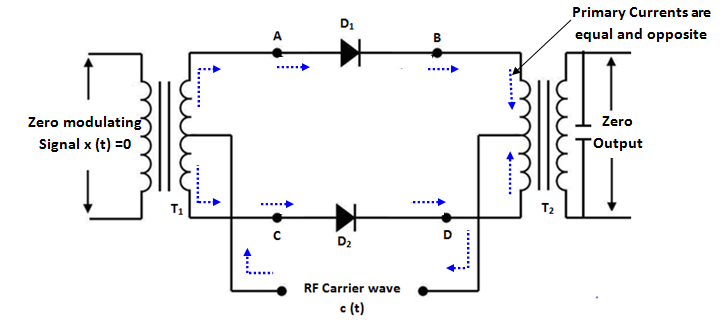Fig.2 : Equivalent circuit in Mode 1 (i)

As shown in the fig.2 , the diodes   D1  and  Dare forward biased and the diodes  D3  and  Dare reverse biased .

We can observe that the direction of currents flowing through the primary windings of output transformer  T2  are equal and opposite to each other .

Therefore, the magnetic fields produced by these currents are equal and opposite and cancel each other .

Hence, the induced voltage in secondary winding is zero . Thus, the carrier is supported in the positive half-cycle .

### (ii) Operation in the Negative half-cycle of Carrier

In this mode also let us assume that the modulating signal is zero .

In the negative half-cycle of the carrier, the diodes D3  and  Dare forward biased and the diodes   D1  and  Dare reverse biased .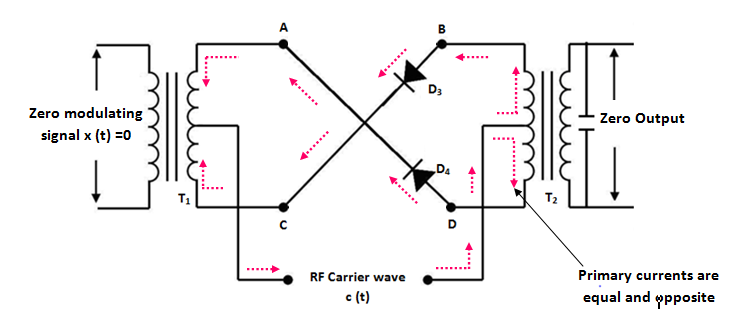Fig 3 : Equivalent circuit of in Mode 1 (ii)

In fig.3 , the currents flowing in the upper and lower halves of the primary winding of Tare again equak and in opposite directions . This cancels the magnetic fields as explained in mode 1 (i) .

Thus, the output voltage in this mode also is zero .

Thus, the carrier is suppressed in the negative half-cycle as well .

It is important to note that the perfect cancellation of the carrier will take place if and only if he characteristics of the diodes are perfectly matched and the centre tap is placed exactly at the centre of the primary transformer T2  .

### Mode 2 : Operation in Presence of Modulating Signal

Now, let us discuss the operation  when RF carrier and modulating signal both are applied .

### (i) Operation in the positive half-cycle of Modulating Signal

As we apply the low frequency modulating signal through the input audio transformer T, there are many cycles of the carrier signal, in the positive half cycle of the modulating signal .

In the positive half-cycle of the carrier, D1  and  Dare ON and secondary of Tis applied as it is across the primary of T2. Hence, during the positive half cycle of carrier, the output of Tis positive as shown in fig.4 (a) .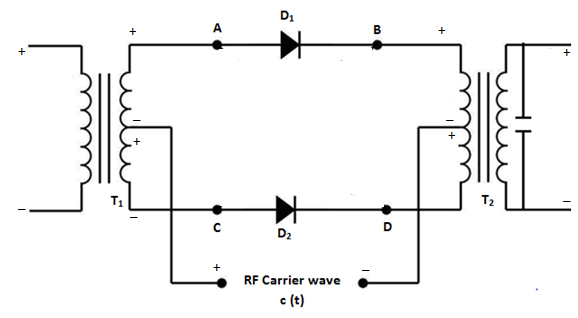Fig 4 (a)

In the negative half-cycle of the carrier,  the diodes D3  and  Dare turned ON and the secondary of  Tis applied in a  reversed manner across the primary of Tas shown in equivalent circuit of fig. (b) .

Thus, the primary voltage of Tis negative and output voltage also becomes negative .

### (ii) Operation in the Negative half-cycle of Modulating Signal

When modulating signal reverses the polarities, the operation of the circuit is same as that in the positive half-cycle discussed earlier .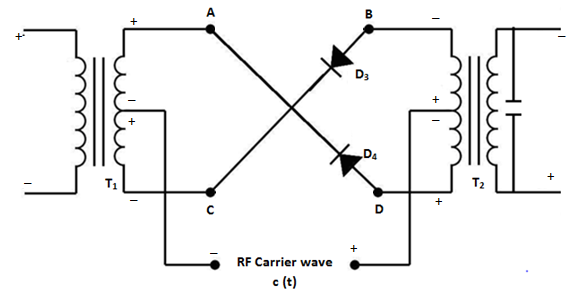Fig 4 (b)

Now, the only difference is that the diode pair  D3 Dwill produce a positive output voltage whereas  D1 D2  will produce a negative output voltage as shown in the waveforms of fig.5 .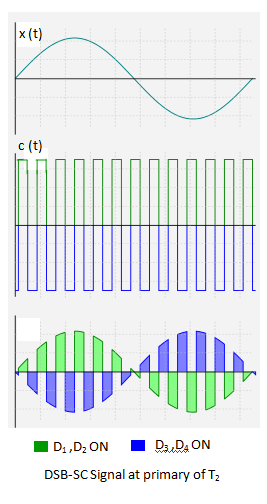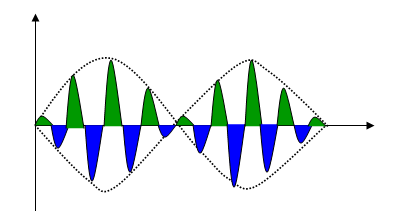DSB-SC output (Output of transformer T2 )

Fig 5

## Analysis of Ring Modulator

From the discussion till now, it is clear that in the positive half cycle of the carrier, the message signal x(t) is multiplied by +1 and in the negative half-cycle of the carrier, x(t) is multiplied by -1 .

Thus, the ring modulator is an ideal form of product modulator and hence it produces the desired DSB-SC output .

The square-wave carrier signal can be represented by the Fourier series as under :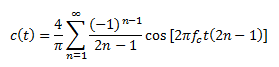hence, the Ring-modulator output is given by :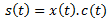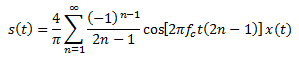It may be noted that there is no output from the modulator at carrier frequency . Therefore, the carrier is entirely eliminated .